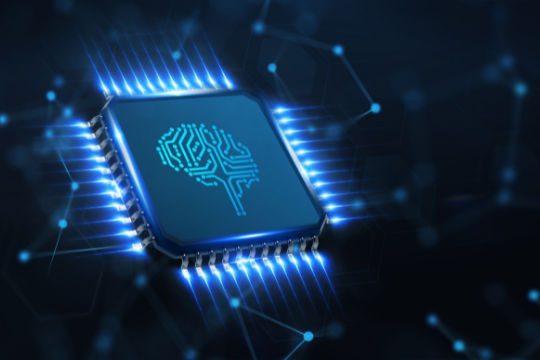# Machine Learning vs. Statistical Learning: Compare & ContrastMachine learning is machine training that provides computers with the ability to learn without being explicitly programmed. Statistical learning involves applying math and statistics to complex problems. Machine learning can be applied to any area where information has been digitized (e.g., images, sound, text) and machine learned models built from basic machine learning algorithms can be used in a wide variety of tasks requiring pattern recognition or predictions.

The following is an overview of machine learning vs statistical learning.

## Machine Learning

In machine learning, machine learners are supposed to tolerate uncertainty or ambiguity; machine learners do not need to be certain of the solution. Machine learning focuses on extracting knowledge from data and includes techniques for concept formation, pattern detection, automated feature selection methodologies such as clustering, regression analysis etc. Automated machine learning includes the following steps:

1. Data collection: Data sets are selected to be used for machine learning; ML algorithms use these data sets
2. Data analysis: It is essential to do an exploratory analysis of the machine learned dataset as it helps in understanding the complexity of machine learning problems at hand and also helps in developing appropriate ML algorithms.
3. Learning & making predictions: Algorithms can help machines learn and make predictions by extracting features from data.
4. Model evaluation: Model performance is evaluated using validation methodologies such as holdout method, cross validation etc.

The machine learning process may involve these steps when this model is used for predictive or classification purposes. Machine learning has a wide scope and huge applications in today’s world.

## Statistical Learning

On the other hand, statistical learning has more focus on data analysis and modeling. The main purpose of statistical learning is to understand patterns in data and aims at providing insights into the data by developing models that explain the underlying process causing these observations.

Unlike machine learners, statistical learners need to be certain of the solution; statistical learners are intolerant of uncertainty.

Statistical learning is not new and has been around for centuries but with advancements in technology it has gained popularity. It has a strong foundation in probability, and machine learning algorithms are based on statistical machine learning techniques.

## A Deeper Understanding of Machine Learning and Statistical Learning

For machine learning, it does not matter if the automation process can answer a question. As long as it can give an accurate estimate with reasonable accuracy, machine learners are happy.

Different ML techniques have different capabilities and carry different advantages; this is the reason machine learning is very popular in many applications today. Machine learned models built from basic algorithms can be used in a wide variety of  tasks requiring pattern recognition or predictions. Statistical machine learning involves applying ML models and concepts to statistical problems where models/algorithms are uncertain about the underlying process causing observations.

Machine learning is not new; it has been around for centuries but with advancements in technology it has gained popularity and its scope of applications have increased very rapidly.

Machine learners (ML) make use of ML algorithms and statistical learning algorithms but statistical learners (SL) make use of simple linear regression (SLR), generalized linear models (GLM) etc. ML problems can be solved via coding (e.g., linear regression) whereas for SL it is easier to solve using simple algorithms that do not require any pre-programming. With statistical learning, we can make use of mathematical models which have stochastic components, such as generalized linear models (GLM).  ML is a branch of computer science, at the heart of which lies algorithms; SL is a field that deals with data analysis and modeling. Machine learning makes use of both supervised and unsupervised machine learning models whereas statistical learning only uses conventional statistical models. ML algorithms are not designed to capture the real-world assumptions that go into creating a statistical model. Statistical learners are concerned with how well their model fits the data rather than what it would look like. ML is more related to computer science and statistical learning is a part of statistics.

## Conclusion

Based on the above discussion we can say that both statistical learning and machine learning are used on different occasions. Machine learning and statistical learning are two different fields where both are based on statistics; however, they differ in several aspects such as methodology of studying data, applications, etc. The scope of machine learners is wider than that of statistical learners since ML has a larger set of algorithms, whereas statistical learners only use traditional statistics. Machine Learning is based on the randomized search technique while statistical learning involves performing regression analysis and building models which allow us to predict future events.

Sunvera Software develops next-level software applications from start-to-finish. Schedule a free 30-minute call with us to discuss your business, or you can give us a call at (949) 284-6300.